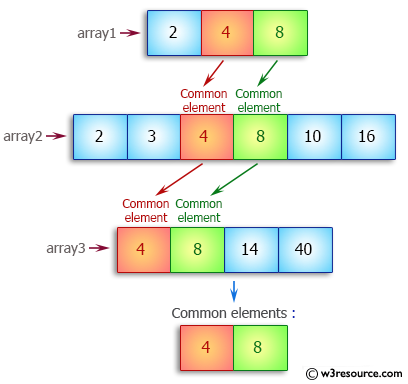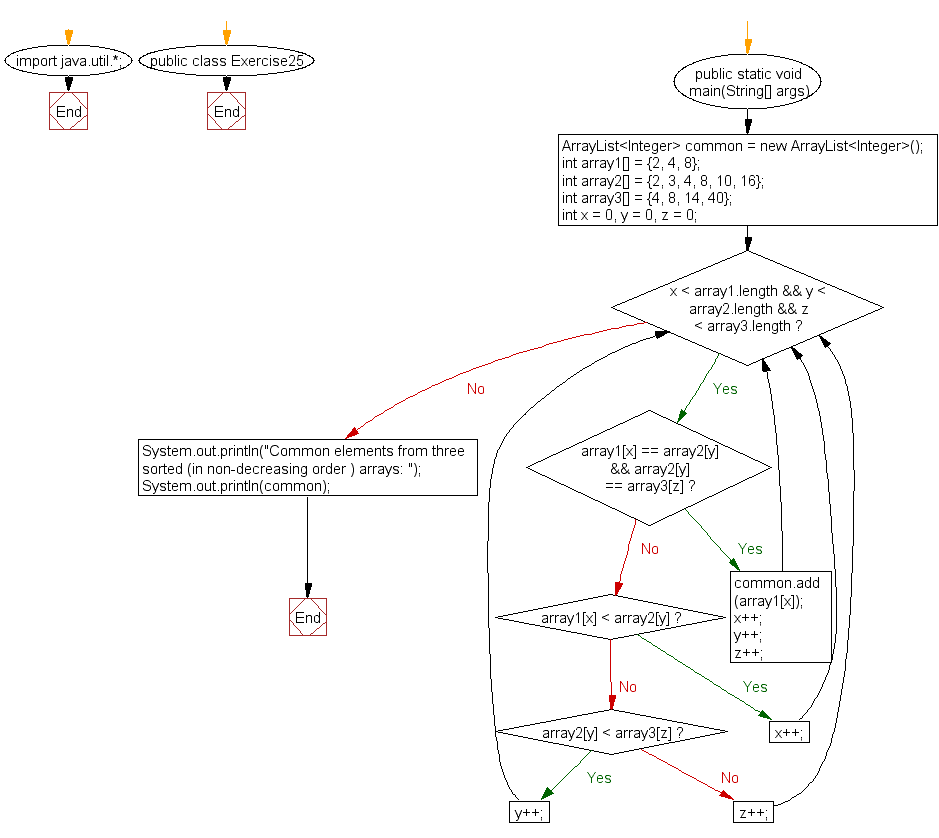﻿ Java exercises: Find common elements from three sorted arrays - w3resource# Java Array Exercises: Find common elements from three sorted arrays

## Java Array: Exercise-25 with Solution

Write a Java program to find common elements from three sorted (in non-decreasing order) arrays.

Pictorial Presentation:Sample Solution:

Java Code:

``````import java.util.*;
public class Exercise25 {
public static void main(String[] args) {
ArrayList<Integer> common = new ArrayList<Integer>();
int array1[] = {2, 4, 8};
int array2[] = {2, 3, 4, 8, 10, 16};
int array3[] = {4, 8, 14, 40};
int x = 0, y = 0, z = 0;
while (x < array1.length && y < array2.length && z < array3.length){
if (array1[x] == array2[y] && array2[y] == array3[z]){
x++;
y++;
z++;
}
else if (array1[x] < array2[y])
x++;
else if (array2[y] < array3[z])
y++;
else
z++;
}
System.out.println("Common elements from three sorted (in non-decreasing order ) arrays: ");
System.out.println(common);
}
}
```
```

Sample Data: array1 = 2, 4, 8
array2 = 2, 3, 4, 8, 10, 16
array3 = 4, 8, 14, 40

Sample Output:

```
Common elements from three sorted (in non-decreasing order ) arrays:
[4, 8]
```

Flowchart:Visualize Java code execution (Python Tutor):

Java Code Editor:

Improve this sample solution and post your code through Disqus

What is the difficulty level of this exercise?

Test your Programming skills with w3resource's quiz.

﻿

## Java: Tips of the Day

countOccurrences

Counts the occurrences of a value in an array.

Use Arrays.stream().filter().count() to count total number of values that equals the specified value.

```public static long countOccurrences(int[] numbers, int value) {
return Arrays.stream(numbers)
.filter(number -> number == value)
.count();
}
```

Ref: https://bit.ly/3kCAgLb